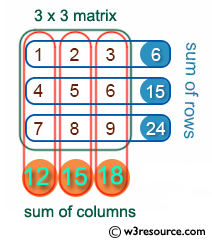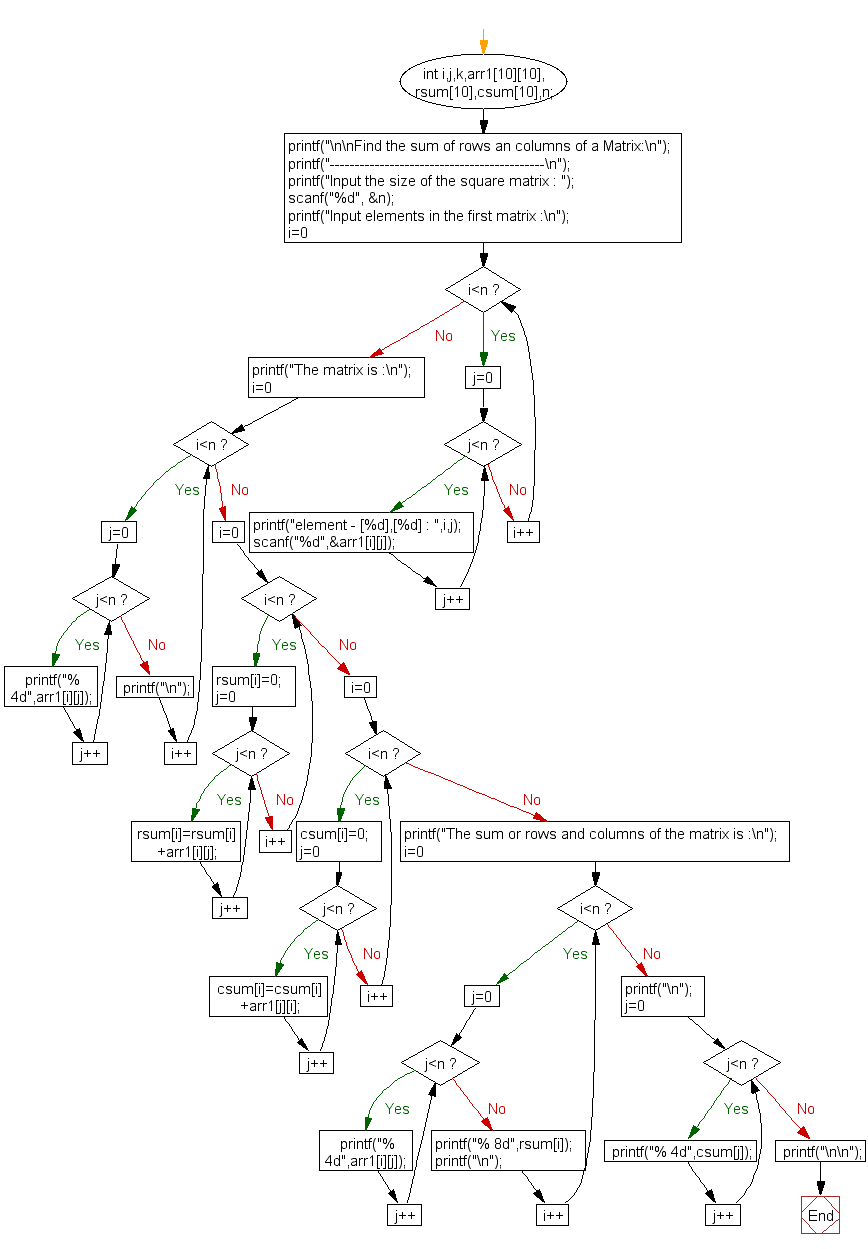﻿ C Program: Find the sum of row an column of a Matrix - w3resource

# C Exercises: Find the sum of rows an columns of a Matrix

## C Array: Exercise-25 with Solution

Write a program in C to find the sum of rows and columns of a matrix.

Visual Presentation:Sample Solution:

C Code:

``````#include <stdio.h>

int main() {
int i, j, k, arr1, rsum, csum, n;

// Display the purpose of the program
printf("\n\nFind the sum of rows and columns of a Matrix:\n");
printf("-------------------------------------------\n");

// Input the size of the square matrix
printf("Input the size of the square matrix : ");
scanf("%d", &n);

// Input elements into the matrix
printf("Input elements in the matrix :\n");
for (i = 0; i < n; i++) {
for (j = 0; j < n; j++) {
printf("element - [%d],[%d] : ", i, j);
scanf("%d", &arr1[i][j]);
}
}

// Display the matrix
printf("The matrix is :\n");
for (i = 0; i < n; i++) {
for (j = 0; j < n; j++) {
printf("% 4d", arr1[i][j]);
}
printf("\n");
}

// Calculate the sum of rows
for (i = 0; i < n; i++) {
rsum[i] = 0;
for (j = 0; j < n; j++) {
rsum[i] = rsum[i] + arr1[i][j];
}
}

// Calculate the sum of columns
for (i = 0; i < n; i++) {
csum[i] = 0;
for (j = 0; j < n; j++) {
csum[i] = csum[i] + arr1[j][i];
}
}

// Display the matrix with row sums
printf("The sum of rows and columns of the matrix is :\n");
for (i = 0; i < n; i++) {
for (j = 0; j < n; j++) {
printf("% 4d", arr1[i][j]);
}
printf("% 8d", rsum[i]); // Print the sum of each row
printf("\n");
}

// Display the column sums
printf("\n");
for (j = 0; j < n; j++) {
printf("% 4d", csum[j]); // Print the sum of each column
}
printf("\n\n");

return 0;
}
```
```

Sample Output:

```Find the sum of rows an columns of a Matrix:
-------------------------------------------
Input the size of the square matrix : 2
Input elements in the first matrix :
element - , : 5
element - , : 6
element - , : 7
element - , : 8
The matrix is :
5   6
7   8
The sum or rows and columns of the matrix is :
5   6      11
7   8      15

12  14
```

Flowchart:C Programming Code Editor:

What is the difficulty level of this exercise?

Test your Programming skills with w3resource's quiz.

﻿